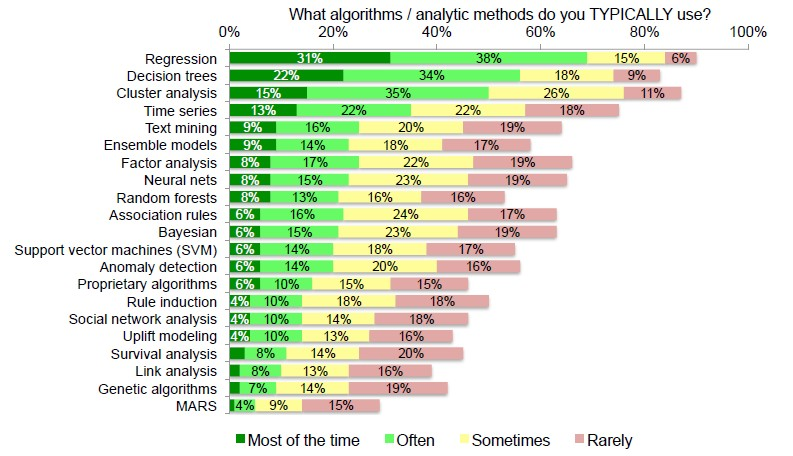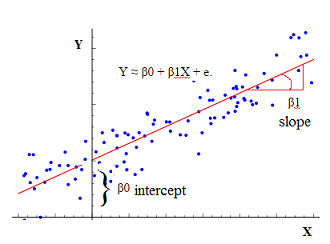# Statistics - Null Hypothesis Significance Testing (NHST)

NHST is a procedure for testing the Null Hypothesis.

It's a binary decision:

• Reject or Retain the Null Hypothesis
• Ie Retain the Null Hypothesis or the alternative hypothesis.

Before starting any experimentation (ie test), two hypothesis are set up:

• The Null hypothesis $H_0$ . There is no relationship between X and Y (nothing is happening, no effects) For example:
• versus the alternative hypothesis $H_0$ . There is some relationship between X and Y. For example:
• r > 0
• a regression. Slope > 0

The game of NHST is to start with the assumption that the Null hypothesis is true.

During an experimentation, we compare:

• an experimental group: the $H_0$ : Null Hypothesis (No difference between the groups)
• and a control group: the $H_A$ : Alternative Hypothesis (Statistically significant difference between the groups)

The “difference” is defined in terms of test statistic:

• Different means (e.g., t-test),
• different variances (e.g., F-test)

The p-value gives the probability that the difference is not only due to the chance.

## Examples

• The new ad placement produces more click-throughs
• This treatment produces better outcomes

## Direction

The test is known :

• as Directional (one tailed test) if the alternative hypothesis predicts the direction of the relationship between X & Y (positive vs. negative))
• Otherwise as non-directional (two tailed test)

Example: Set up for a Non-directional for regression:

• Ho. Slope = 0
• Ha. Slope <> 0

## Lifecyle

Steps:

• Initial assumption: The Null Hypothesis $H_0$ is true
• Calculate all our statistics
• Calculate the probability of observing data with these characteristics, given that initial assumption that $H_0$ is true. That gives the p-value.

$$p = P(D | H_0)$$

• Reassess the Initial assumption: If p is very low, then Reject $H_0$ , else Retain $H_0$

It's sort of odd and backwards. Very rarely assumptions are made that predict no relationship between two variables. It's rare to look for no relationship. It's a little bit weird and backwards.

### multiple regression

• Assume the null hypothesis is true (Slope = 0), then no one sample should have a very high of very low p-value (Ie p <0.5).
• Conduct a study
• Calculate the statistics:
• If I obtained a very high B, reject the NULL hypothesis.

## Four possible outcomes

Four boxes, four outcomes.

Either the Null is true or is false.

Experimenter Decision
Retain H0 Reject H0
H0 true Correct
Decision
Type I error
(False alarm)
H0 false Type II error
(Miss)
Correct
Decision

False Alarm: claiming that it works when it facts it really doesn't. Sometimes that happens. You might get an initial result that looks good and it say it works. But then as you do more research, get more representative samples, better assessments, you may find out that it doesn't work.

Miss: There is really an effect out there and you just missed it. For any reasons: poor assessment, not enough subjects, no random representative sample.

Never made a conclusion on one study because they are prone to this errors.

 Do not reject $H_0$ Reject $H_0$ Correct Decision 1 -­ $alpha$ Type 1 error $alpha$ Type 2 error $beta$ Correct Decision 1 -­ $beta$ The power of test

## Statistics / Equation

Most NHST's statistics are essentially ratios.

They are basically, what did you observe relative to what do you expect just due to chance.

That was standard error. How much sampling error are we going to get, just due to chance.

## Problems

### Biased by sample size

We'll get a significant result almost all the time if we just obtain a really large sample (Big sample size, Big N). See t-value formula.

If NHST results are reported, it is important to also report effect size

### Arbitrary decision rule

• The cut-off value (alpha) is arbitrary
• p < .05 is considered standard but still arbitrary
• Problems arise when p is close to .05 but not less than .05

### Yokel local test

• Many researchers use NHST because it’s the only approach they know
• NHST encourages weak hypothesis testing

### Error prone

If p then q
Not q
Therefore, not p

If the null hypothesis is correct, then these data can not occur
The data have occurred
Therefore, the null hypothesis is false


If the null hypothesis is correct, then these data are highly unlikely
These data have occurred
Therefore, the null hypothesis is highly unlikely


If a person plays football, then he or she is probably not a professional player
This person is a professional player
Therefore, he or she probably does not play football



## Alternative

Alternative to a Null Hypothesis Significance Test:

• Model comparison
• Bayesian inference

Discover MoreData Mining - Algorithms

An is a mathematical procedure for solving a specific kind of problem. For some data mining functions, you can choose among several algorithms. Algorithm Function Type Description Decision...Logical Data Modeling - Binary Relation

A binary relation is a relationship between two elements that is implemented via a binary function. Binary relations are used in many branches of mathematics to model concepts like: order relation...Statistical - Inference

Methods for drawing conclusions a population from sample data Two key methods Hypothesis tests (significance tests) Confidence intervals E.g., t-test – enables inferences population beyond our...Statistics - (F-Statistic|F-test|F-ratio)

The NHST anova statistic test is an F-test or F-ratio. It's what you observe in the numerator relative to what you would expect just due to chance in the denominator. The f statistic is the statistic...Statistics - (Student's) t-test (Mean Comparison)

The t-test is a test that compares means. NHST can be conducted yielding to a p-value Effect Size can be calculated like in multiple regression. Confidence Interval around the mean can also be...Statistics - (Univariate|Simple|Basic) Linear Regression

A Simple Linear regression is a linear regression with only one predictor variable (X). Correlation demonstrates the relationship between two variables whereas a simple regression provides an equation...Statistics - (dependent|paired sample) t-test

A dependent t-test is appropriate when: we have the same people measured twice. the same subject are been compared (ex: Pre/Post Design) or two samples are matched at the level of individual subjects...Statistics - (t-value|t-statistic)

The (t-value|t-statistic) is a test statistic. In NHST, it is essentially a ratio of what we observed relative to what we would expect just due to chance. Each t-value has corresponding p-value depending...Statistics - Analysis of variance (Anova)

Anova is just a special case of multiple regression. There're many forms of ANOVA. It's a very common procedure in basic statistics. Anova is more Appropriate when: there's true independent variable...Statistics - Confidence Interval

The definition of a confidence interval says that under repeated experiments 95% of the time this confidence interval will contain the true statistic (mean, ...). if we started the whole experiment over...